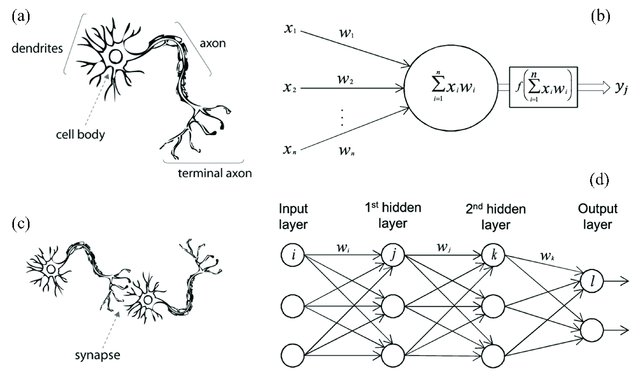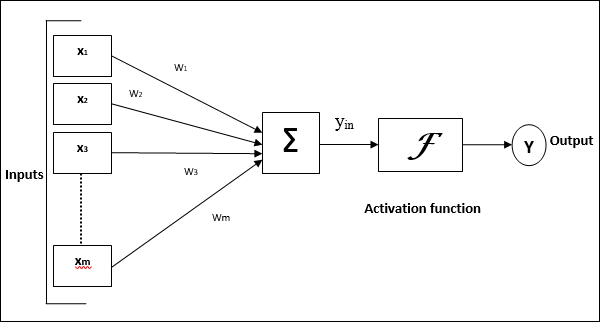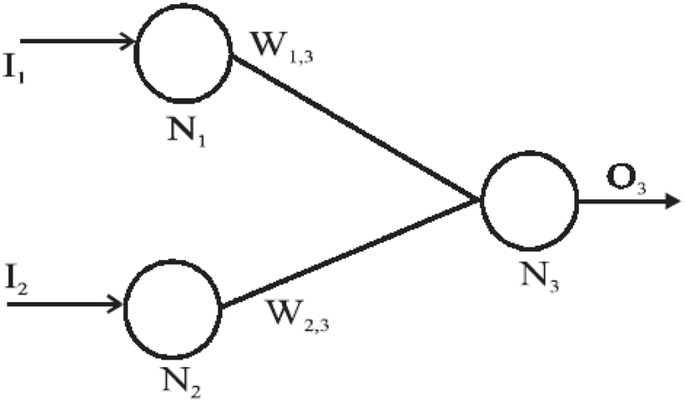# Artificial Neural Network – Part 1

01/06/2020

## Introduction

In this series of posts, I would like to create an artificial neural network from scratch. In this post, I will like to give an introduction to human neuron and a comparison to ANN. Using a single-layered perceptron model, I will try to solve a linearly separable problem.

Artificial neural networks are the backbone of artificial intelligence and deep learning. They are versatile and scalable, making them ideal for tackling complex tasks like image classification, speech recognition, recommendation systems and natural language processing.

## Biological vs Artificial Neurons

We are trying to imitate the functioning of the brain. Although we don’t exactly know what exactly happens in the brain, we have a basic understanding. We are trying to approximate it using mathematical functions.
The human brain has billions of neurons. In humans, neurons are a network of 100 million neurons and 60 trillion connections. If each neuron is approximated to a computing unit, this cannot be replicated currently by computers.

In the human brain, a neuron consists of different parts:
Soma: The cell body (Approximated to the neuron in ANN)
Dendrites: Input to the cell (Approximated to the input)
Synopses: It is the weight assigned to the input (Approximated to the weight or slope)
Axon: Output to a neuron (Approximated to the final output)

### What happens in a neuron?

When a neuron receives signals from different dendrites, the signals are cumulated. In a simplified understanding, if this cumulated signal is greater than a certain value, it passes these signals to the next neuron. Neuron, when it communicates with neighbouring neurons, it uses electrochemical reactions which are approximated to a mathematical function called the activation function.

In humans, neurons have computing powers as well as cognition powers. Also, the human brain neural network is plastic, that means, some neurons die, new neurons are created, the synapses change, etc.### How do we approximate it to an ANN

In an Artificial neuron, the soma is approximated to the neuron. The dendrites are the input function, and the synapses are the weights and Axon is approximated to an output. A representation of a neuron (also called TLU) is below:Activation function: In biological systems, the neurons transmit signals after it reaches some threshold potential. The below figure shows how the neuron transmits the signal only after the signal is greater than the threshold potential. In ANN, this is mathematically represented using an activation function.The perceptron is the simplest ANN architecture. A perceptron contains a single layer of TLU(Threshold Logical Units). The input and outputs are numbers, and each of the input has a weight. A weighted sum of the inputs is computed (z), and then an activation function is applied to get the result (y).
$z = w_1 \times x_1 + w_2 \times x_2 ... w_n \times x_n$ In the current example, the step function is the activation function. If the data is mutually separable, a step function is sufficient for classification problems.
$step(z) = \left\{ \begin{array}{ll} 0 & z< \theta \\ 1 & z\geq \theta \\ \end{array} \right.$

Also, ANN’s are models from where simple business understandable rules cannot be generated, which creates a reluctance for its widespread use when other explainable models can be used.

A perceptron model has four steps:

Step 1: Set initial weights $w_1, w_2 ... w_n$ and threshold $\theta$ (bias). We generally take small values between [-0.5, 0.5]
Step 2: Identify the activation function
Step 3: Weight training: Weight is updated based on error: The weight correction is done based on the error. The weight in node i is the previous weight plus an additional correction. This correction is a product of learning rate, input value and error. $w_i(p+1) = w_i(p) + \delta w_i(p)$ $\delta w_i(p) = \alpha \times x_i(p) \times e(p)$

where $w_i(p)$ is the weight at step p correction and $\alpha$ is the learning rate.

Step 4: Repeat till stopping criterion

Even with such a simple model, we can train the model to perform logical computations like and-gate. The input and output for an and-gate are as follows:

input 1 input 2 output
0 0 0
0 1 0
1 0 0
1 1 1

A single layer single TLU is taken to solve this problem.### Step 1:

Randomly I am choosing weights of $w_{1,3} = 0.3$, $w_{2,3} = -0.1$, $\theta = 0.2$ and $\alpha = 0.1$

### Step 2:

The activation function is the step function which is described above.

### Step 3:

For the above weights, The output for the four scenarios is:

Output for epoch 1
input_a input_b w_a w_b output error delta_a delta_b
0 0 0.3 -0.1 0 0 0.1x0x0 = 0 0.1x0x0 = 0
0 1 0.3 -0.1 0 0 0.1x0x0 = 0 0.1x1x0 = 0
1 0 0.3 -0.1 0 -1 0.1x1x-1 = -0.1 0.1x0x-1 = 0
1 1 0.2 -0.1 1 1 0.1x1x1 = 0.1 0.1x1x1 = 0.1

In the third iteration, when the input 1 is 1 and input 2 is 0, the error is -1. The corrections in weights is as follows:
weight a
$\delta w_{1,3}(3) = \alpha \times x_1(3) \times e(3) = 0.1\times 1\times -1 = -0.1$
$w_{1,3}(4) = w_{1,3}(3) + \delta w_{1,3}(3)$

weight b
$\delta w_{2,3}(3) = \alpha \times x_2(3) \times e(3) = 0.1\times 0\times -1 = -0$
$w_{2,3}(4) = w_{2,3}(3) + \delta w_{2,3}(3)$

### Step 4

One such iteration is called an epoch. For the optimal neural network, we should run different epochs until the errors (also called as the cost function) is zero. As this is a linearly separable case, the stopping criterion is that the error is zero.
Neural Network and-gate
epoch input_a input_b w_a w_b output y error delta_a delta_b
1 0 0 0.3 -0.1 0 0 0 0.1x0x0 = 0 0.1x0x0 = 0
1 0 1 0.3 -0.1 0 0 0 0.1x0x0 = 0 0.1x1x0 = 0
1 1 0 0.3 -0.1 0 1 -1 0.1x1x-1 = -0.1 0.1x0x-1 = 0
1 1 1 0.2 -0.1 1 0 1 0.1x1x1 = 0.1 0.1x1x1 = 0.1
2 0 0 0.3 0.0 0 0 0 0.1x0x0 = 0 0.1x0x0 = 0
2 0 1 0.3 0.0 0 0 0 0.1x0x0 = 0 0.1x1x0 = 0
2 1 0 0.3 0.0 0 1 -1 0.1x1x-1 = -0.1 0.1x0x-1 = 0
2 1 1 0.2 0.0 1 0 1 0.1x1x1 = 0.1 0.1x1x1 = 0.1
3 0 0 0.3 0.1 0 0 0 0.1x0x0 = 0 0.1x0x0 = 0
3 0 1 0.3 0.1 0 0 0 0.1x0x0 = 0 0.1x1x0 = 0
3 1 0 0.3 0.1 0 1 -1 0.1x1x-1 = -0.1 0.1x0x-1 = 0
3 1 1 0.2 0.1 1 1 0 0.1x1x0 = 0 0.1x1x0 = 0
4 0 0 0.2 0.1 0 0 0 0.1x0x0 = 0 0.1x0x0 = 0
4 0 1 0.2 0.1 0 0 0 0.1x0x0 = 0 0.1x1x0 = 0
4 1 0 0.2 0.1 0 0 0 0.1x1x0 = 0 0.1x0x0 = 0
4 1 1 0.2 0.1 1 1 0 0.1x1x0 = 0 0.1x1x0 = 0

After 4th epoch, the error is zero. The final weights are $w_{1,3} = 0.2$ and $w_{2,3} = 0.1$

In the second blog, I would be discussing using ANN for the titanic problem and comparing with logistic regression. Back propagation will be explained.

### More reading material

1. Biological vs artificial neurons: https://www.tutorialspoint.com/artificial_neural_network/artificial_neural_network_basic_concepts.htm
2. Basics implimentation: Géron, A., 2019. Hands-On Machine Learning with Scikit-Learn, Keras, and TensorFlow: Concepts, Tools, and Techniques to Build Intelligent Systems. O’Reilly Media.
3. And gate implimentation: Fausett, L., 1994. Fundamentals of neural networks: architectures, algorithms, and applications. Prentice-Hall, Inc..
4. Reference: Negnevitsky, M., 2005. Artificial intelligence: a guide to intelligent systems. Pearson education.